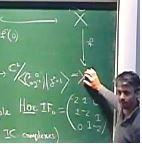# Geordie Williamson

University of Sydney
Visit:
Tuesday, May 30, 2017 to Thursday, June 1, 2017
Lectures:

Lecture 1 (5/30): Algebraic representations

This will be an introduction to the theory of algebraic representations. I will discuss the representation theory of SL_2, and general reductive algebraic groups, recalling the fundamental Steinberg tensor product and restriction theorems. I will then turn to Lusztig's character formula and its status.

Lecture 2 (5/31): Constructible sheaves

I will discuss the geometric Satake equivalence and Finkelberg-Mirkovic conjecture. This provides a conceptually satisfying setting in which to understand Lusztig's conjecture. Understand Lusztig's conjecture for a fixed prime leads to subtle questions concerning torsion in intersection cohomology. I will discuss what is known and what remains to be understood.Williamson.pdf